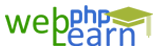Apply for Zend Framework Certification Training

# Mysql

```Hi viewers we have many Comparison operator in Mysql like < , > , = , <= , => .
Using These We can perform Comparison While selection of Data .
Eg:-
1) Let say you want to Select Age range student from 19 to 25 for Job purpose.

SELECT * FROM students WHERE (Age >= 19 AND Age <= 25);

2) Let say you want to Select Age range student from 19 to 23 or 25 to 28 for Job purpose.

SELECT * FROM students WHERE (Age >= 19 AND Age <= 23) OR (Age >= 25 AND Age <= 28);

3) We can use here Between Also  To get the same Date

SELECT * FROM students where age between(19,23);

4) We Can also use here IN  in this query

SELECT * from students where age in(19,20,21,22,23);

5) SELECT DATEDIFF("2017-01-01", "2016-12-24");```

`{{questionlistdata.blog_question_description}}`
`{{answer.blog_answer_description  }}`